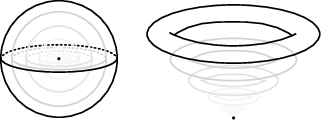Martin David Palmer -- Preprint -- Homological stability for moduli spaces of disconnected submanifolds, II - Symmetric diffeomorphism groups and parametrised connected sum
Papers and preprints
 Stability for moduli spaces of manifolds with conical singularities (v1: 2018, v2: 2020) arXiv: abs — pdf v2 — v1 Abstract The homology of configuration spaces of point-particles in manifolds has been studied intensively since the 1970s; in particular it is known to be stable if the underlying manifold is connected and open. Closely related to configuration spaces are moduli spaces of manifolds with marked points, and in [Tillmann, 2016] this relation was used to show that the homology of these moduli spaces is also stable as the number of marked points goes to infinity. Since a disc neighbourhood of a marked point may be viewed as the cone on its boundary sphere, we may think of marked points as inessential conical singularities in the underlying manifold. In this paper, we prove stability for the homology of moduli spaces of manifolds with conical singularities, allowing more general – essential – singularities. This is deduced as a special case of a more general homological stability result for classifying spaces of symmetric diffeomorphism groups of manifolds, with respect to parametric connected sum, an operation generalising ordinary connected sum and surgery (including Dehn surgery). The key input for the proof is stability for the homology of moduli spaces of disconnected submanifolds [Palmer, 2018], which generalises configurations of point-particles to configurations of higher-dimensional submanifolds. Along the way, we extend this result further to homology with polynomially twisted coefficients and configurations of labelled submanifolds. Note: The original working title of this paper (the title of its first version on the ArXiv) was "Homological stability for moduli spaces of disconnected submanifolds, II – Symmetric diffeomorphism groups and parametrised connected sum". It is a continuation of my work in the paper Homological stability for moduli spaces of disconnected submanifolds, I. Click here for the notes of a talk that I gave, based on the results of this paper, on 26 July 2018 in Salvador, Brazil, at the satellite meeting Braid groups, configuration spaces and homotopy theory of the 2018 ICM. NB: these are very abbreviated notes intended for a 25–minute talk, so some statements have been simplified.